# AVL Trees Notes for GATE

AVL Tree is an essential topic under one of the most important chapters of Computer Science i.e. Data Structure. And, when it comes to a competitive examination like GATE, you have to dive deep into this topic to understand it thoroughly. In this article, we have comprised all the pointers related to the AVL Tree. We hope these notes for CSE topics will help you understand this topic in a better way.

# What is an AVL Tree?

The term AVL tree was introduced by Adelson-Velsky and Landis. It is a balanced binary search tree and is the first data structure like this. In the AVL tree, the heights of the subtree cannot be more than one for all nodes.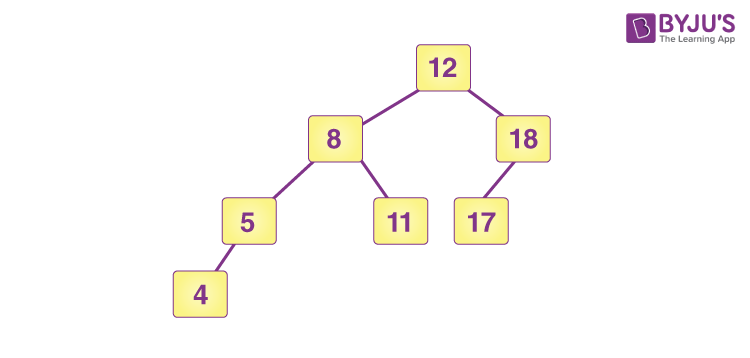The above picture is of an AVL tree. Here, it is clearly visible that the heights of the left and right subtrees are equal to, or less than one.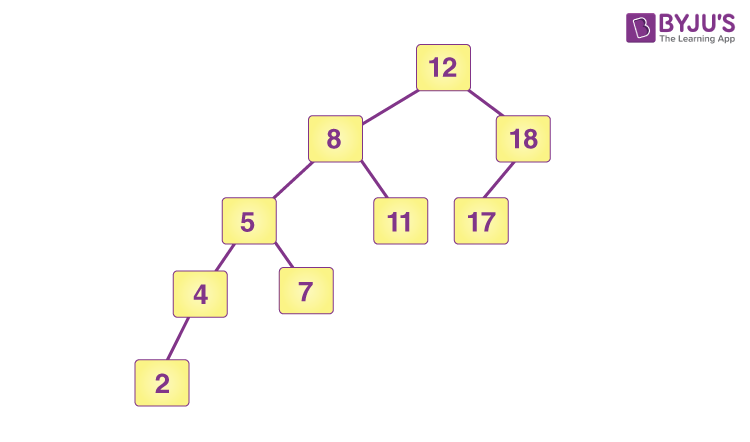The above tree is not an AVL tree. And, the reason is simple. Here, the heights of the left and right subtrees are higher than 1.

## Balance Factor in AVL Tree

In the AVL tree, the term balance factor is very important.

Balance Factor = height(left-subtree) – height(right – subtree)

The balanced factor should be -1, 0 or +1. Otherwise, the tree will be considered an unbalanced tree.

Example Of AVL Tree & Balance Factor: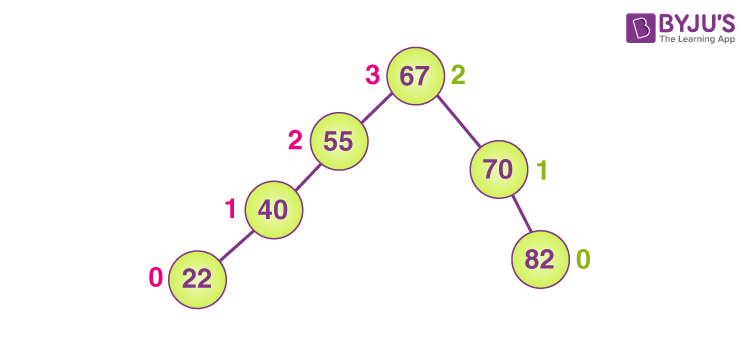In the above example, the height of the left subtree is 3 and the height of the right subtree is 2. That means the balance factor is <=1 therefore the tree is supposed to be balanced.

## Why Do We Need an AVL Tree?

To reduce the issue of time complexity in a binary search tree, the AVL tree was introduced by Adelson-Velski & Landis. It is a self-balancing tree that helps in reducing the complexity issue.

### Operations on AVL Tree

The AVL tree is a balancing binary tree, and therefore it follows the same operations we perform in the binary search tree.

1. Insertion
2. Deletion

Insertion: The process of insertion is the same as it is executed in the binary search tree. However, there are chances that it may point to a violation in the AVL tree property, and the tree may require balancing. To balance a tree we can apply rotations.

Deletion: The process of deletion is the same as it is executed in a binary search tree. It can affect the balance factor of the tree, therefore, we need to utilize different types of rotations to balance the tree.

## AVL Rotation

• Left rotation
• Right rotation
• Left-Right rotation
• Right-Left rotation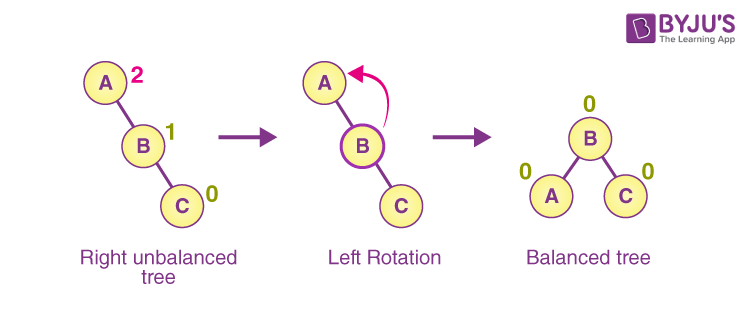1. Left Rotation: When we perform insertion at the left subtree, then it is a left rotation.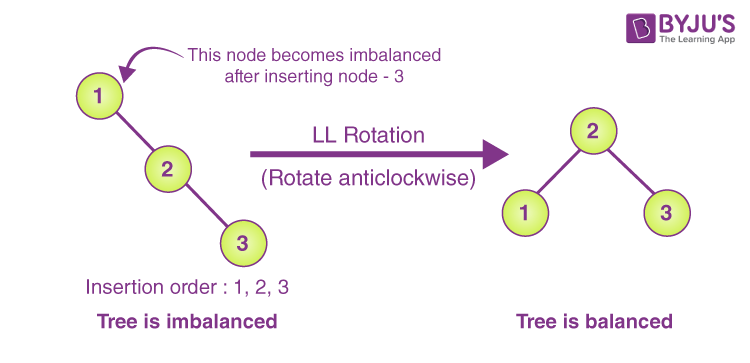1. Right Rotation: When we perform insertion at the right subtree, then it is a right rotation.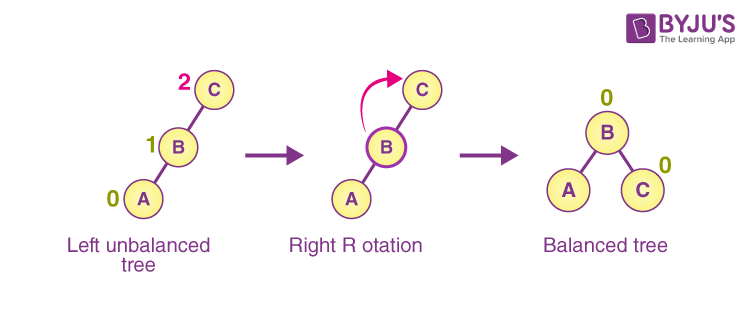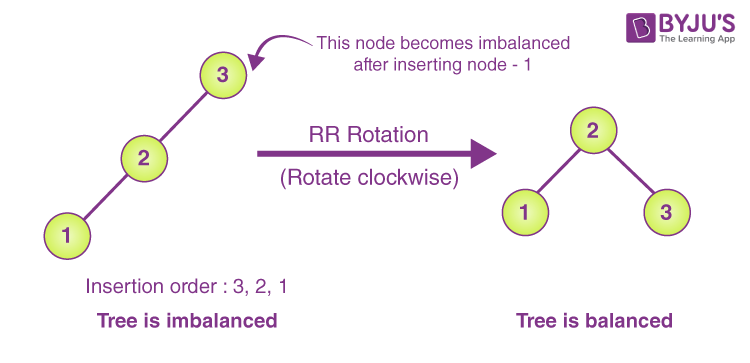1. Left-Right Rotation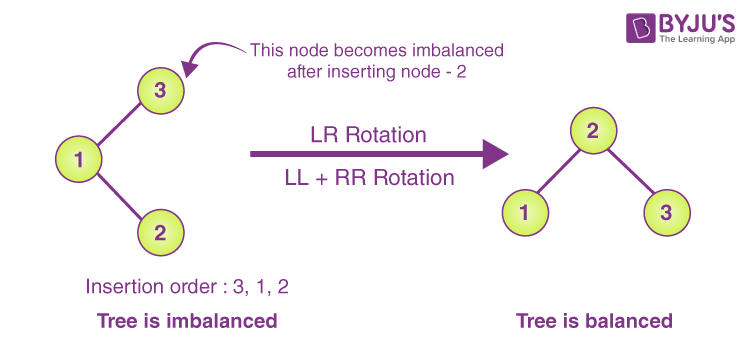1. Right-Left Rotation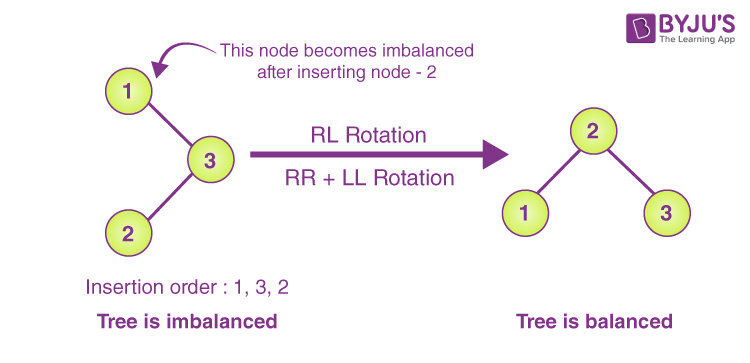Figure: Right-Left Rotation

## Time Complexity in AVL Tree

 Algorithm Average case Worst case Space o(n) o(n) Search o(log n) o(log n) Insert o(log n) o(log n) Delete o(log n) o(log n)

## Practice Problem – AVL Tree

Q. What is the maximum height of any AVL-tree with 7 nodes? Assume that the height of a tree with a single node is 0.

• (A) 2
• (B) 3
• (C) 4
• (D) 5

Keep learning and stay tuned to get the latest updates on GATE Exam along with GATE Eligibility CriteriaGATE 2023GATE Admit CardGATE Syllabus for CSE (Computer Science Engineering)GATE CSE NotesGATE CSE Question Paper, and more.

Also Explore,